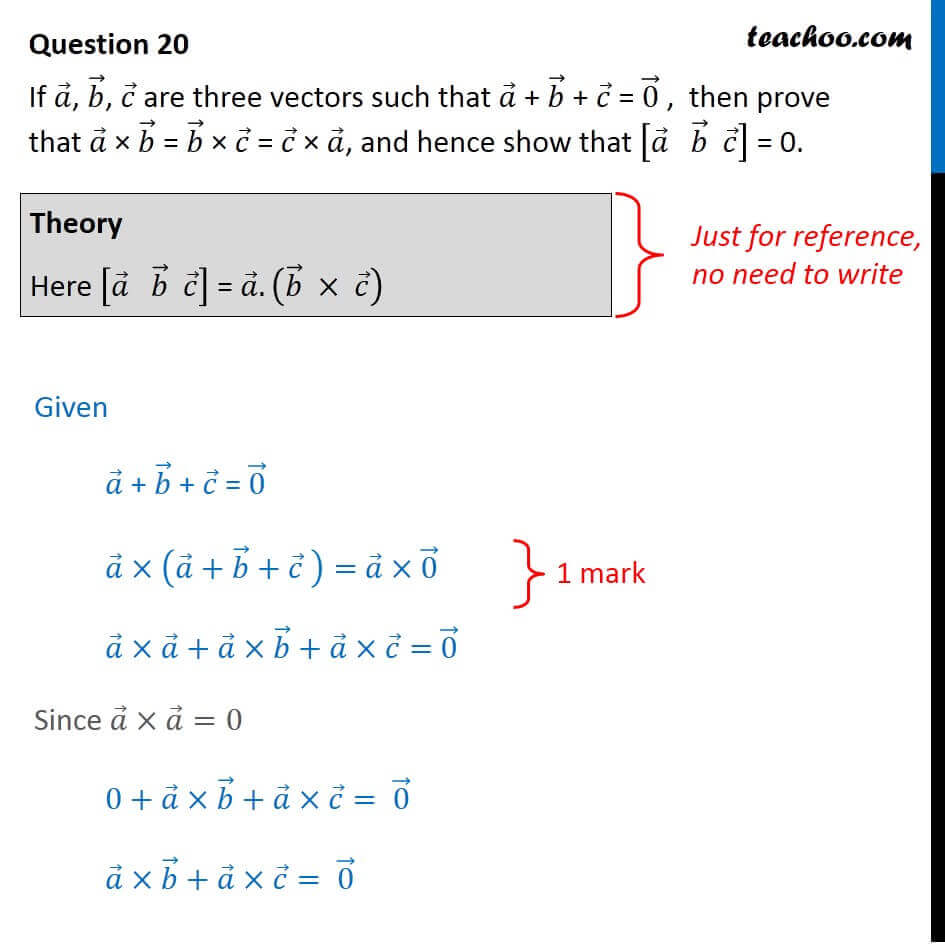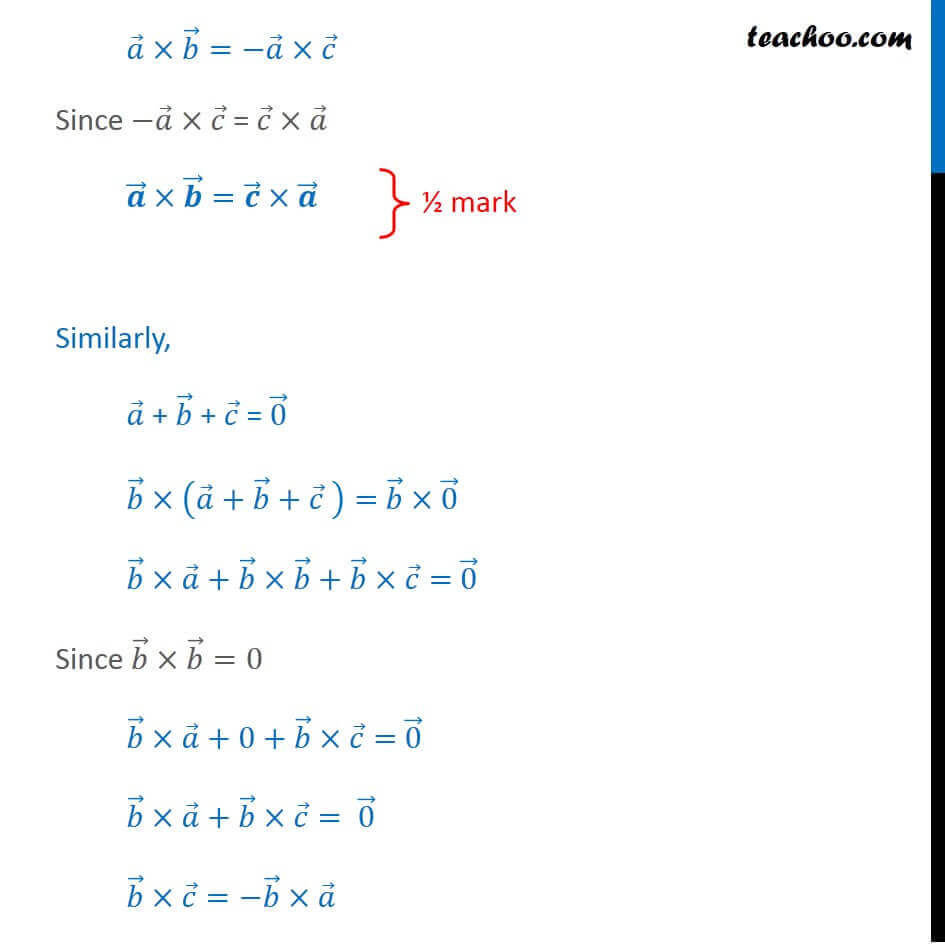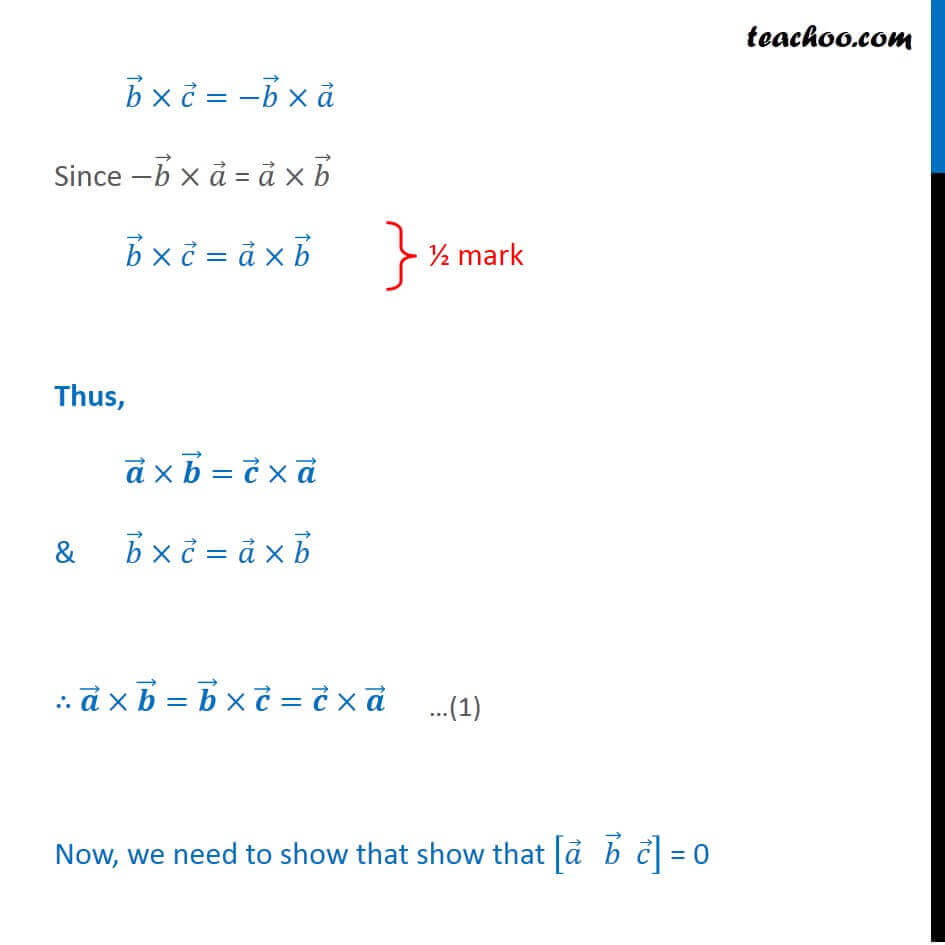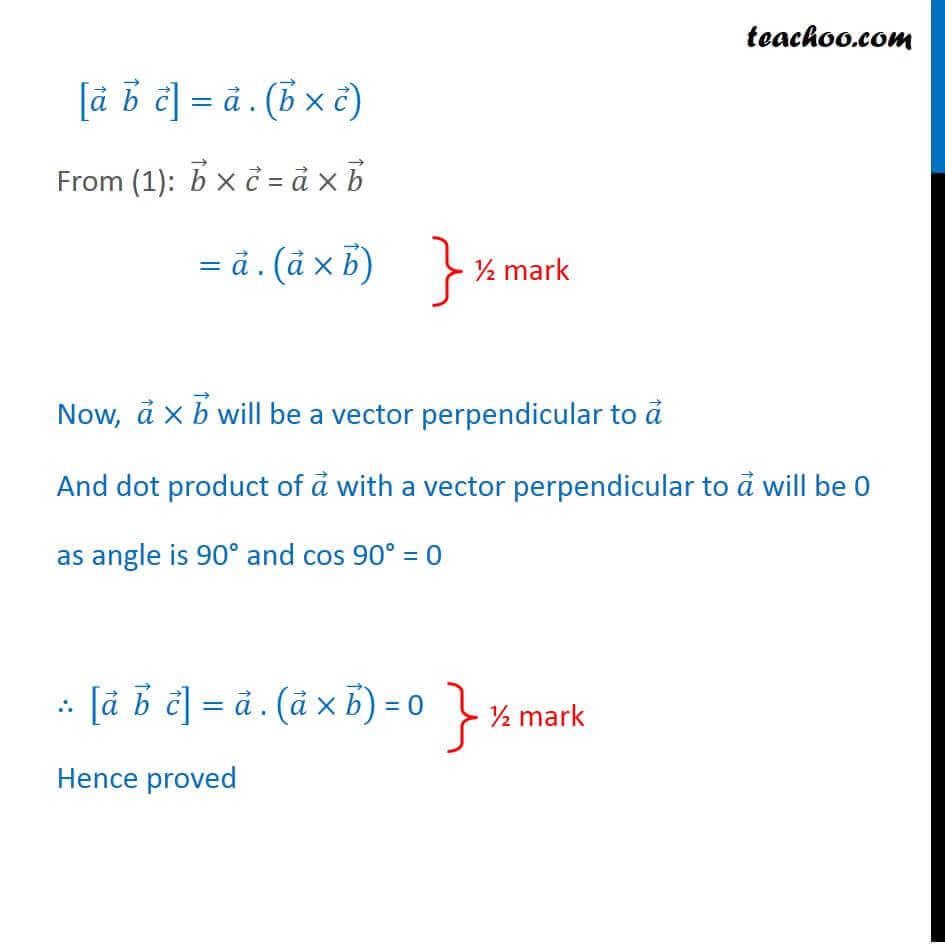CBSE Class 12 Sample Paper for 2018 Boards

Class 12
Solutions of Sample Papers and Past Year Papers - for Class 12 Boards

### If a, b, c are three vectors such that a + b + c = 0 ,  then prove that a × b = b × c = c × a, and hence show that [a b c] = 0.

This is a question of CBSE Sample Paper - Class 12 - 2017/18.Introducing your new favourite teacher - Teachoo Black, at only ₹83 per month

### Transcript

Question 20 If 𝑎 ⃗, 𝑏 ⃗, 𝑐 ⃗ are three vectors such that 𝑎 ⃗ + 𝑏 ⃗ + 𝑐 ⃗ = 0 ⃗ , then prove that 𝑎 ⃗ × 𝑏 ⃗ = 𝑏 ⃗ × 𝑐 ⃗ = 𝑐 ⃗ × 𝑎 ⃗, and hence show that [𝑎 ⃗" " 𝑏 ⃗" " 𝑐 ⃗ ] = 0. Theory Here [𝑎 ⃗" " 𝑏 ⃗" " 𝑐 ⃗ ] = 𝑎 ⃗.(𝑏 ⃗ × 𝑐 ⃗ ) Given 𝑎 ⃗ + 𝑏 ⃗ + 𝑐 ⃗ = 0 ⃗ 𝑎 ⃗×(𝑎 ⃗+𝑏 ⃗+𝑐 ⃗ )= 𝑎 ⃗×0 ⃗ 𝑎 ⃗×𝑎 ⃗+𝑎 ⃗×𝑏 ⃗+𝑎 ⃗×𝑐 ⃗= 0 ⃗ Since 𝑎 ⃗×𝑎 ⃗=0 " " 0+𝑎 ⃗×𝑏 ⃗+𝑎 ⃗×𝑐 ⃗=" " 0 ⃗ 𝑎 ⃗×𝑏 ⃗+𝑎 ⃗×𝑐 ⃗=" " 0 ⃗ 𝑎 ⃗×𝑏 ⃗=−𝑎 ⃗×𝑐 ⃗ Since −𝑎 ⃗×𝑐 ⃗ = 𝑐 ⃗×𝑎 ⃗ 𝒂 ⃗×𝒃 ⃗=𝒄 ⃗×𝒂 ⃗ Similarly, 𝑎 ⃗ + 𝑏 ⃗ + 𝑐 ⃗ = 0 ⃗ 𝑏 ⃗×(𝑎 ⃗+𝑏 ⃗+𝑐 ⃗ )= 𝑏 ⃗×0 ⃗ 𝑏 ⃗×𝑎 ⃗+𝑏 ⃗×𝑏 ⃗+𝑏 ⃗×𝑐 ⃗= 0 ⃗ Since 𝑏 ⃗×𝑏 ⃗=0 𝑏 ⃗×𝑎 ⃗+0+𝑏 ⃗×𝑐 ⃗= 0 ⃗ 𝑏 ⃗×𝑎 ⃗+𝑏 ⃗×𝑐 ⃗=" " 0 ⃗ 𝑏 ⃗×𝑐 ⃗=−𝑏 ⃗×𝑎 ⃗ 𝑏 ⃗×𝑐 ⃗=−𝑏 ⃗×𝑎 ⃗ Since −𝑏 ⃗×𝑎 ⃗ = 𝑎 ⃗×𝑏 ⃗ 𝑏 ⃗×𝑐 ⃗=𝑎 ⃗×𝑏 ⃗ Thus, 𝒂 ⃗×𝒃 ⃗=𝒄 ⃗×𝒂 ⃗ & 𝑏 ⃗×𝑐 ⃗=𝑎 ⃗×𝑏 ⃗ ∴ 𝒂 ⃗×𝒃 ⃗=𝒃 ⃗×𝒄 ⃗=𝒄 ⃗×𝒂 ⃗ Now, we need to show that show that [𝑎 ⃗" " 𝑏 ⃗" " 𝑐 ⃗ ] = 0 [𝑎 ⃗ 𝑏 ⃗ 𝑐 ⃗ ]=𝑎 ⃗ . (𝑏 ⃗×𝑐 ⃗ ) From (1): 𝑏 ⃗×𝑐 ⃗ = 𝑎 ⃗×𝑏 ⃗ =𝑎 ⃗ . (𝑎 ⃗×𝑏 ⃗ ) Now, 𝑎 ⃗×𝑏 ⃗ will be a vector perpendicular to 𝑎 ⃗ And dot product of 𝑎 ⃗ with a vector perpendicular to 𝑎 ⃗ will be 0 as angle is 90° and cos 90° = 0 ∴ [𝑎 ⃗ 𝑏 ⃗ 𝑐 ⃗ ]=𝑎 ⃗ . (𝑎 ⃗×𝑏 ⃗ ) = 0 Hence proved